# Polarizing Prism

## Polarizing Prism

a type of optical prism used as a linear polarizer—that is, linearly polarized optical radiation can be obtained by means of it. It usually consists of two or more triangular prisms, at least one of which is cut from an optically anisotropic crystal.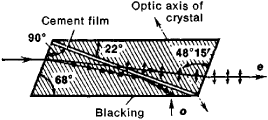Figure 1. Nicol prism: (o) ordinary ray, (e) extraordinary ray. The hatching indicates the direction of the optic axes of the crystals in the plane of the drawing. The directions of the vibrations of the electric vectors of the light waves are indicated on the rays by arrows (for vibrations in the plane of the figure) and dots (for vibrations perpendicular to the plane of the figure). The blacking on the lower face of the prism absorbs the ordinary ray, which is totally reflected at the plane of the cement. The cement is Canada balsam.

Polarizing prisms are constructed in such a way that the radiation transmitted through them must be able to penetrate the inclined interface between two media. The conditions of refraction for the components of the light beam that are polarized in two mutually perpendicular planes differ sharply at this interface. In particular, the conditions for total internal reflection can be satisfied for one of these components at the interface. As a result, only the other component is transmitted through the prism. Examples of this type of prism are the widely used Nicol prism, which is often called simply a Nicol (Figure 1), and the Foucault prism (Figure 2). In these prisms, the extraordinary ray e is transmitted and the ordinary ray o is intercepted—it is absorbed or deflected. Such prisms are called single-image prisms. Double-image prisms transmit both mutually perpendicular, linearly polarized components of the original beam by spatially separating them. Polarizing prisms are usually made of Iceland spar, CaCO3, which is transparent for wavelengths of 0.2 to 2 microns (μ), or of crystalline quartz, SiO2, which is transparent for wavelengths of 0.185 to 3.5 μ.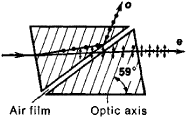Figure 2. The shortened polarizing prism of Foucault with an air film; the symbols are the same as in Figure 1

The triangular prisms of which single image polarizing prisms are made are often cemented together with a transparent substance whose refractive index n is close to the mean of the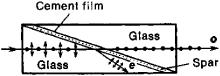Figure 3. Linear polarizer (polarizing prism) made of glass and Iceland spar; the dots in the layer of spar indicate that the spar’s optic axis is perpendicular to the plane of the figure; the other symbols are the same as in Figure 1

refractive indexes of the ordinary (no) and extraordinary (ne) rays. Such substances as Canada balsam, glycerin, castor oil, and linseed oil are used as the cementing agents. The parts of many polarizing prisms are separated by an air film rather than glue; this technique reduces absorption losses when the radiation densities are high and offers a number of advantages for work in the ultraviolet region of the spectrum. In some cases, the parts of a prism may be separated by a layer of fused quartz. Use is also made of polarizing prisms in which a crystalline plate is cemented between two glass prisms whose refractive index is close to the higher refractive index of the crystal (Figure 3). In such polarizing prisms, the ordinary ray is transmitted, and the extraordinary ray is reflected. In order for one of the rays to undergoFigure 4. Limiting angles of incidence I1 and I2 of rays on a Glan-Thompson prism. The symbols associated with the rays are the same as in Figure 1. Canada balsam, which gives an aperture for complete polarization ∊=I1 + I2 = 27.5°, or linseed oil, for which ∊ = 41°, is used as the cement. The angle α is equal to 76.5°.

total internal reflection at the cement interface, there are selected certain values of the refracting angles of the triangular prisms and, generally, certain orientations of the optic axes of the crystals from which the prisms are cut. Such reflection occurs if the angles of incidence of the rays on the polarizing prism do not exceed some limiting angles I1 and I2. This fact is illustrated by the Glan-Thompson prism in Figure 4. The sum I1+I2, which is called the aperture of the prism for complete polarization, is of great importance in work involving polarizing prisms in converging beams of radiation.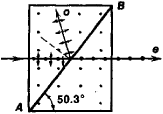Figure 5. Glan prism: (AB) air film. The dots on the two triangular prisms indicate that the optic axes of the prisms are perpendicular to the plane of the figure. The symbols associated with the rays are the same as in Figure 1.

In polarizing prisms with slanted faces, such as Nicol and Foucault prisms, the transmitted ray undergoes a parallel displacement. For this reason, when the prism is rotated about the ray, the ray also rotates. This and other shortcomings of such prisms are absent in polarizing prisms that have the shape of right-angled parallelepipeds, such as the Glan-Thompson prism, the Glan prism (Figure 5), the Glazebrook prism (Figure 6), and the Franck-Ritter prism (Figure 7).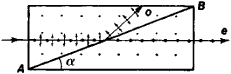Figure 6. Glazebrook prism. The symbols associated with the rays are the same as in Figure 1. When Canada balsam is used as the cement in the plane AB, the angle α = 12.1°; when linseed oil is used, α = 14°; and when glycerin is used, α = 17.3°. The dots indicate that the optic axes of the crystals of the two right-angle prisms are perpendicular to the plane of the figure.

The most widely used double-image polarizing prisms include the Rochon, Sénarmont, and Wollaston prisms (Figure 8). In the Rochon and Sénarmont prisms, the ordinary ray does not undergo a change of direction, but the extraordinary ray is deviated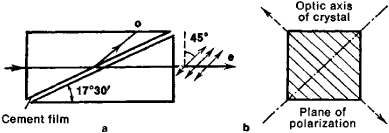Figure 7. Franck-Ritter prism: (a) side view, (b) view along the path of the ray. The optic axes of the crystalline right-angle prisms are directed at an angle of 45° to the plane of (a) and at an angle of 90° to the plane of the vibrations of the electric vector of the extraordinary ray — that is, to the plane of polarization of the ray. The cement is Canada balsam.

by an angle θ. The value of θ is about 5° to 6° and is strongly dependent on the wavelength of the light: θ= (no — ne)tan α, where a is the refracting angle of the triangular prisms. A Wollaston prism produces a doubled angle of divergence 2θ, or about 10°; for perpendicular incidences, the deviations of the rays are symmetric. The Wollaston prism is used in polarizing photometers, spectrophotometers, and polarimeters. The angle α in polarizing prisms made of Iceland spar is close to 30°; for prisms made of crystalline quartz it is about 60°.Figure 8. Double-image prisms: (a) Rochon prism, (b) Sénarmont prism, (c) Wollaston prism, (d) prism made of Iceland spar and glass, (e) Abbe prism. The hatching indicates the direction of the optic axes of the crystals in the plane of the figure. The dots mean that the optic axis is perpendicular to the plane of the figure. The arrows and dots associated with the rays indicate the directions of the vibrations of the electric vector.

Polarizing prisms are generally characterized by a small aperture for complete and relatively large size. They must be handled with care but are essentially free of chromatic aberration. Moreover, they are irreplaceable for work in the ultraviolet region of the spectrum and in strong fluxes of optical radiation, and they permit the obtaining of uniformly polarized beams whose degree of polarization differs from unity by only ~10-5.

V. S. ZAPASSKII

Site: Follow: Share:
Open / Close